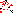# MINLPLib

### A Library of Mixed-Integer and Continuous Nonlinear Programming Instances

#### Instance: bearing

 Formatsⓘ ams gms mod nl osil Primal Bounds (infeas ≤ 1e-08)ⓘ 1.95173322 p1 ( gdx sol ) (infeas: 3e-14)1.95168396 p2 ( gdx sol ) (infeas: 7e-10) Other points (infeas > 1e-08)ⓘ 1.93618060 p3 ( gdx sol ) (infeas: 5e-07)1.92826666 p4 ( gdx sol ) (infeas: 9e-07)1.91339147 p5 ( gdx sol ) (infeas: 9e-07) Dual Boundsⓘ 1.95173322 (ANTIGONE)1.09632259 (BARON)1.86055753 (COUENNE)1.95173322 (LINDO)1.94733263 (SCIP) Referencesⓘ Siddall, James N, Optimal Engineering Design: Principles and Applications, Marcel Dekker, New York, 1982.Deb, Kalyanmoy and Goyal, Mayank, Optimizing Engineering Designs Using a Combined Genetic Search. In Bäck, Thomas, Ed, Proceedings of the Seventh International Conference on Genetic Algorithms, 1997, 521-528.Coello Coello, Carlos A, Treating Constraints as Objectives for Single-Objective Evolutionary Optimization, Engineering Optimization, 32:3, 2000, 275-308. Sourceⓘ GAMS Model Library model bearing Applicationⓘ Hydrostatic Thrust Bearing Design Added to libraryⓘ 31 Jul 2001 Problem typeⓘ NLP #Variablesⓘ 13 #Binary Variablesⓘ 0 #Integer Variablesⓘ 0 #Nonlinear Variablesⓘ 12 #Nonlinear Binary Variablesⓘ 0 #Nonlinear Integer Variablesⓘ 0 Objective Senseⓘ min Objective typeⓘ linear Objective curvatureⓘ linear #Nonzeros in Objectiveⓘ 2 #Nonlinear Nonzeros in Objectiveⓘ 0 #Constraintsⓘ 12 #Linear Constraintsⓘ 3 #Quadratic Constraintsⓘ 4 #Polynomial Constraintsⓘ 3 #Signomial Constraintsⓘ 0 #General Nonlinear Constraintsⓘ 2 Operands in Gen. Nonlin. Functionsⓘ vcpower log10 log Constraints curvatureⓘ indefinite #Nonzeros in Jacobianⓘ 38 #Nonlinear Nonzeros in Jacobianⓘ 28 #Nonzeros in (Upper-Left) Hessian of Lagrangianⓘ 32 #Nonzeros in Diagonal of Hessian of Lagrangianⓘ 6 #Blocks in Hessian of Lagrangianⓘ 2 Minimal blocksize in Hessian of Lagrangianⓘ 1 Maximal blocksize in Hessian of Lagrangianⓘ 11 Average blocksize in Hessian of Lagrangianⓘ 6.0 #Semicontinuitiesⓘ 0 #Nonlinear Semicontinuitiesⓘ 0 #SOS type 1ⓘ 0 #SOS type 2ⓘ 0 Infeasibility of initial pointⓘ 1.008e+05 Sparsity JacobianⓘSparsity Hessian of Lagrangianⓘ```\$offlisting
*
*  Equation counts
*      Total        E        G        L        N        X        C        B
*         13       10        1        2        0        0        0        0
*
*  Variable counts
*                   x        b        i      s1s      s2s       sc       si
*      Total     cont   binary  integer     sos1     sos2    scont     sint
*         14       14        0        0        0        0        0        0
*  FX      0
*
*  Nonzero counts
*      Total    const       NL      DLL
*         41       13       28        0
*
*  Solve m using NLP minimizing objvar;

Variables  x1,x2,x3,x4,objvar,x6,x7,x8,x9,x10,x11,x12,x13,x14;

Equations  e1,e2,e3,e4,e5,e6,e7,e8,e9,e10,e11,e12,e13;

e1..    10000*objvar - 10000*x7 - 10000*x8 =E= 0;

e2.. -1.42857142857143*x4*x6 + 10000*x8 =E= 0;

e3.. 10*x7*x9 - 0.00968946189201592*(x1**4 - x2**4)*x3 =E= 0;

e4.. 143.3076*x10*x4 - 10000*x7 =E= 0;

e5.. 3.1415927*(0.001*x9)**3*x6 - 6e-6*x3*x4*x13 =E= 0;

e6.. 101000*x12*x13 - 1.57079635*x6*x14 =E= 0;

e7.. log10(0.8 + 8.112*x3) - 10964781961.4318*x11**(-3.55) =E= 0;

e8..  - 0.5*x10 + x11 =E= 560;

e9..    x1 - x2 =G= 0;

e10.. 0.0307*sqr(x4) - 0.3864*sqr(0.0062831854*x1*x9)*x6 =L= 0;

e11..    101000*x12 - 15707.9635*x14 =L= 0;

e12.. -(log(x1) - log(x2)) + x13 =E= 0;

e13.. -(sqr(x1) - sqr(x2)) + x14 =E= 0;

* set non-default bounds
x1.lo = 1; x1.up = 16;
x2.lo = 1; x2.up = 16;
x3.lo = 1; x3.up = 16;
x4.lo = 1; x4.up = 16;
x6.lo = 1; x6.up = 1000;
x7.lo = 0.0001;
x8.lo = 0.0001;
x9.lo = 1;
x10.up = 50;
x11.lo = 100;
x12.lo = 1;
x13.lo = 0.0001;
x14.lo = 0.01;

* set non-default levels
x1.l = 6;
x2.l = 5;
x3.l = 6;
x4.l = 3;
x6.l = 1000;
x7.l = 1.6;
x8.l = 0.3;
x10.l = 50;
x11.l = 600;

Model m / all /;

m.limrow=0; m.limcol=0;
m.tolproj=0.0;

\$if NOT '%gams.u1%' == '' \$include '%gams.u1%'

\$if not set NLP \$set NLP NLP
Solve m using %NLP% minimizing objvar;

```

Last updated: 2021-03-11 Git hash: 66559cbc